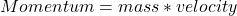A child with a mass of 23 kg rides a bike with a mass of 5.5 kg at a velocity of 4.5 m/s to the south. Compare the momentum of the child wit

Question

A child with a mass of 23 kg rides a bike with a mass of 5.5 kg at a velocity of 4.5 m/s to the south. Compare the momentum of the child with the momentum of the bike

in progress 0
6 months 2021-07-21T16:00:19+00:00 1 Answers 39 views 0

Explanation:

Given the following data;

Mass of child = 23 kg

Mass of bike = 5.5 kg

Velocity = 4.5 m/s

Momentum can be defined as the multiplication (product) of the mass possessed by an object and its velocity. Momentum is considered to be a vector quantity because it has both magnitude and direction.

Mathematically, momentum is given by the formula;To find the momentum of each of them;

I. Momentum of the child

Momentum C = mass * velocity

Momentum C = 23 * 4.5

Momentum C = 103.5 Kgm/s

II. Momentum of the bike

Momentum B = mass * velocity

Momentum B = 5.5 * 4.5

Momentum B = 24.75 Kgm/s

Hence, we can deduce from the calculations that the momentum of the child is greater than that of the bike because of the higher mass possessed by the child.